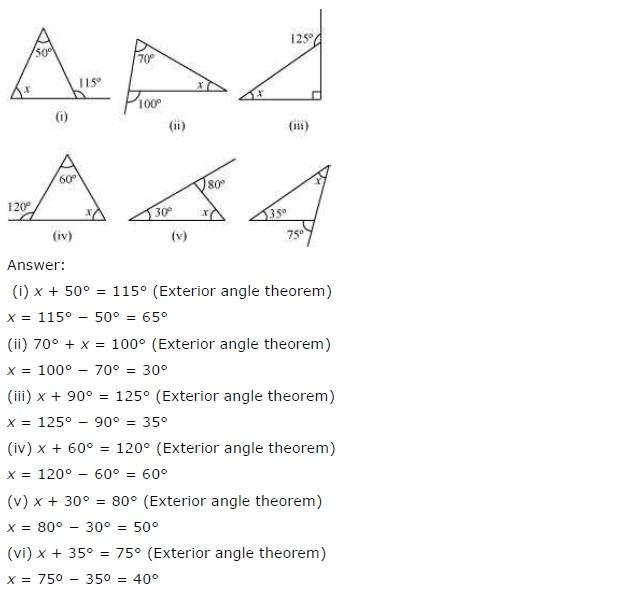# Class 7 Maths The Triangle and Its Properties Exercise 6.2

NCERT Solutions Class-7 Maths chapter-6 Triangle and its properties Exercise-6.2 is prepared by academic team of pw all the questions of NCERT text book are solved step by step with proper and detail solutions explaining each and every questions . For More and additional questions of CBSE class 7 maths you can go to class 7 maths sections. NCERT class 7 Maths Solutions is the best way to enhanced your mathematics skill. And pw practice worksheet & question bank will help you a lot .

## NCERT Solutions class 7 Maths Chapter-6 Triangle and its properties

### Solutions of Chapter Triangle and its properties Exercise-6.2

Question 1:

Find the value of the unknown exterior angles x in the following diagrams: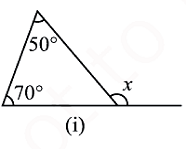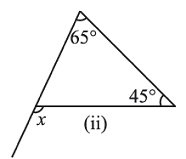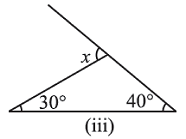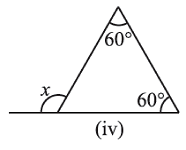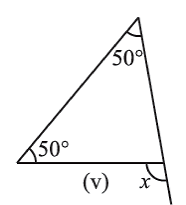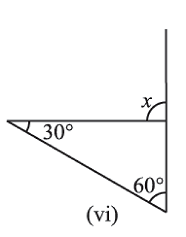(i) It is given in the question that,

1st interior angle = 50o and 2nd interior angle = 70o

Note: According to exterior angle theorem,

The measure of an exterior angle of a triangle is equal to the sum of the measure of the two non-adjacent interior angles of the triangle.

Exterior angle = x

Sum of interior angles = 50o + 70o

Thus, using exterior angle theorem,

x = 50o + 70o

x = 120o

∴ The value of x is 120o

(ii) It is given in the question that,

1st interior angle = 65o and 2nd interior angle = 45o

Note: According to exterior angle theorem,

The measure of an exterior angle of a triangle is equal to the sum of the measure of the two non-adjacent interior angles of the triangle.

Exterior angle = x

Sum of interior angles = 65o + 45o

Using, exterior angle theorem, we have

x = 65o + 45o

x = 110o

Hence, the value of x is 110o

(iii) It is given in the question that,

1st interior angle = 30o and 2nd interior angle = 40o

Note: According to exterior angle theorem,

The measure of an exterior angle of a triangle is equal to the sum of the measure of the two non-adjacent interior angles of the triangle.

Exterior angle = x

Sum of interior angles = 30o + 40o

Using exterior angle theorem, we have

x = 30o + 40o

x = 70o

Hence, the value of x is 70o

(iv) It is given in the question that,

1st interior angle = 60o and 2nd interior angle = 60o

Note: According to exterior angle theorem,

The measure of an exterior angle of a triangle is equal to the sum of the measure of the two non-adjacent interior angles of the triangle.

Exterior angle = x

Sum of interior angles = 60o + 60o

Using exterior angle theorem, we have

x = 60o + 60o

x = 120o

Hence, the value of x is 120o

(v) It is given in the question that,

1st interior angle = 50o and 2nd interior angle = 50o

Note: According to exterior angle theorem:

The measure of an exterior angle of a triangle is equal to the sum of the measure of the two non-adjacent interior angles of the triangle.

Exterior angle = x

Sum of interior angles = 50o + 50o

Using exterior angle theorem, we have

x = 50o + 50o

x = 100o

Hence, the value of x is 100o

(vi) It is given in the question that,

1st interior angle = 30o and 2nd interior angle = 60o

Note: According to exterior angle theorem:

The measure of an exterior angle of a triangle is equal to the sum of the measure of the two non-adjacent interior angles of the triangle.

Exterior angle = x

Sum of interior angles = 30o + 60o

Using, exterior angle theorem, we have

x = 30o + 60o

x = 90o

Hence, the value of x is 120o

Question 2:

Find the value of the unknown interior angle x in the following figures: